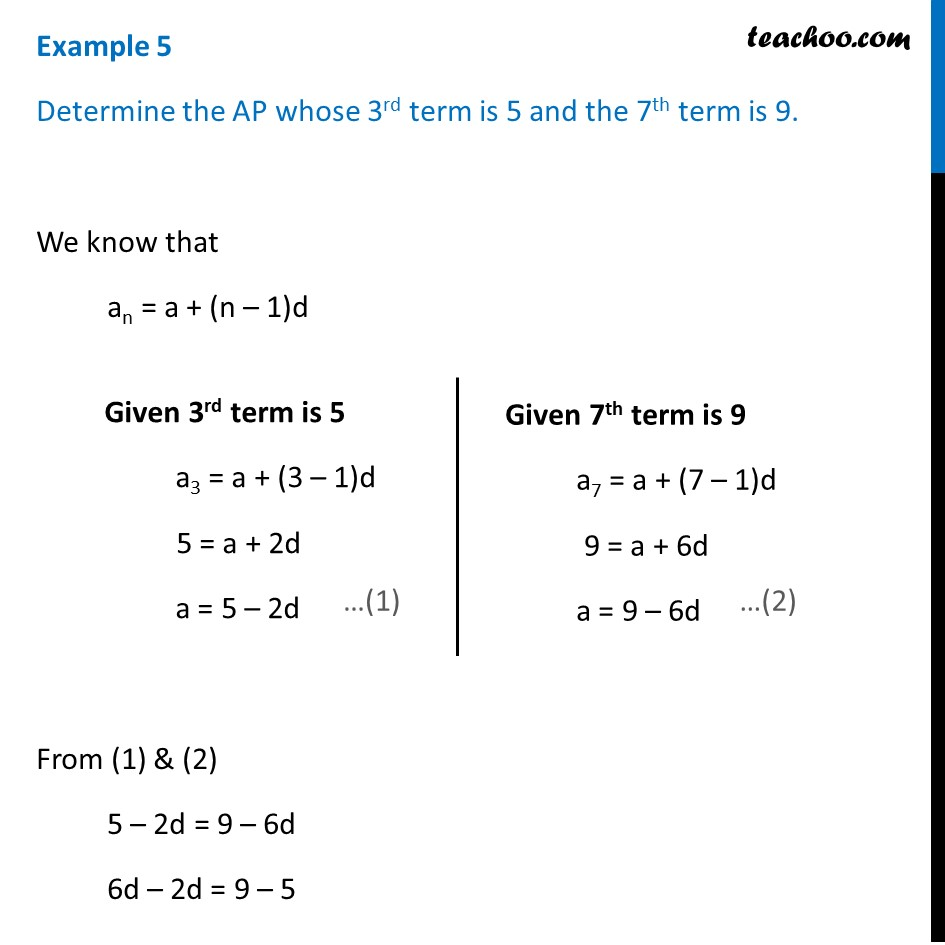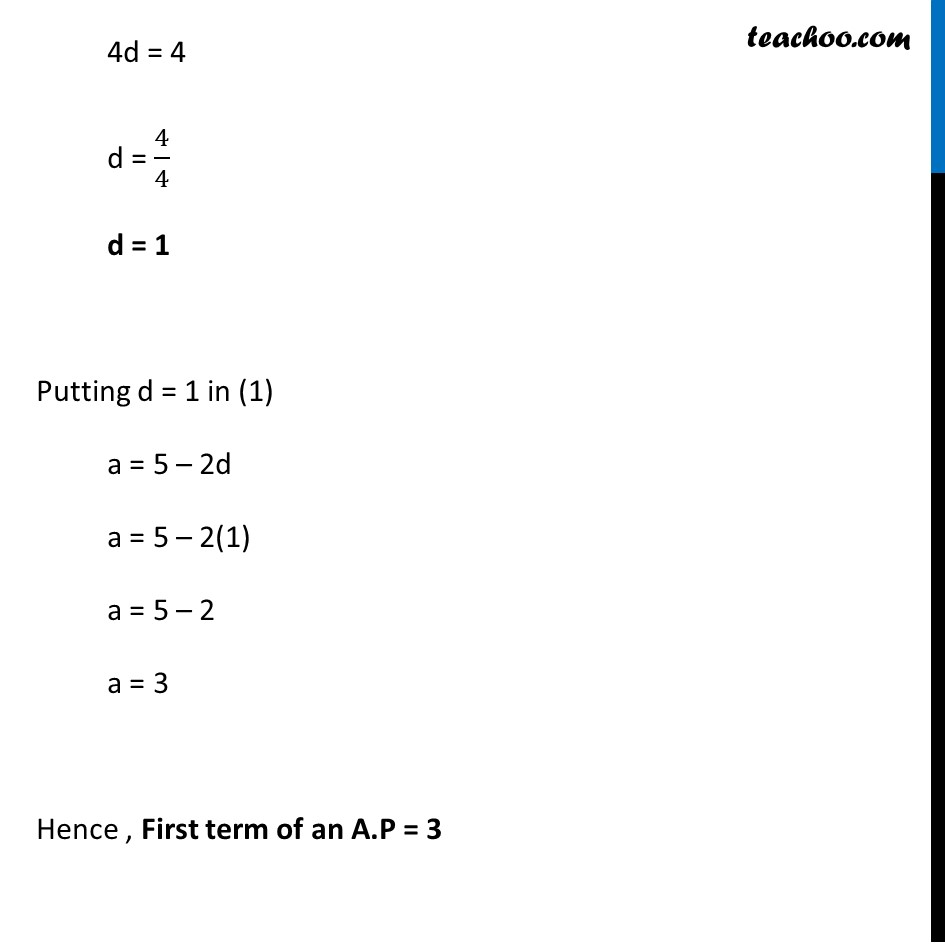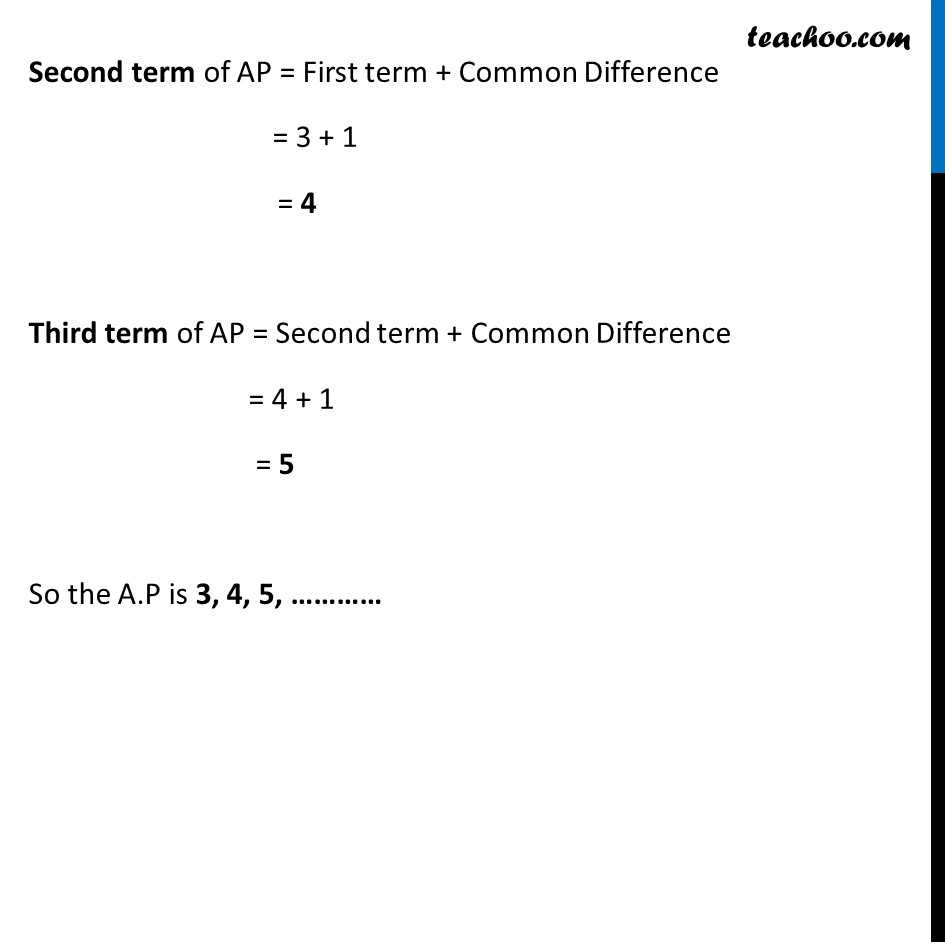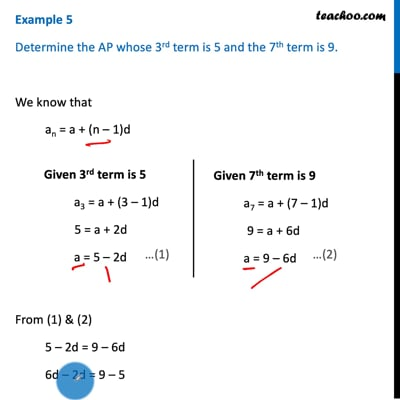Finding AP

Chapter 5 Class 10 Arithmetic Progressions
Concept wiseThis video is only available for Teachoo black users

Introducing your new favourite teacher - Teachoo Black, at only ₹83 per month

### Transcript

Example 5 Determine the AP whose 3rd term is 5 and the 7th term is 9. We know that an = a + (n – 1)d From (1) & (2) 5 – 2d = 9 – 6d 6d – 2d = 9 – 5 Given 3rd term is 5 a3 = a + (3 – 1)d 5 = a + 2d a = 5 – 2d Given 7th term is 9 a7 = a + (7 – 1)d 9 = a + 6d a = 9 – 6d 4d = 4 d = 4/4 d = 1 Putting d = 1 in (1) a = 5 – 2d a = 5 – 2(1) a = 5 – 2 a = 3 Hence , First term of an A.P = 3 Second term of AP = First term + Common Difference = 3 + 1 = 4 Third term of AP = Second term + Common Difference = 4 + 1 = 5 So the A.P is 3, 4, 5, …………